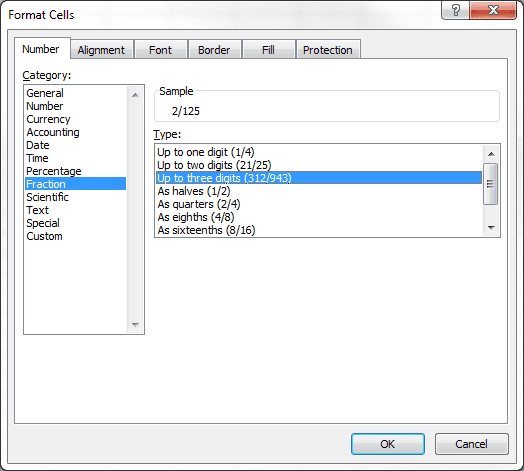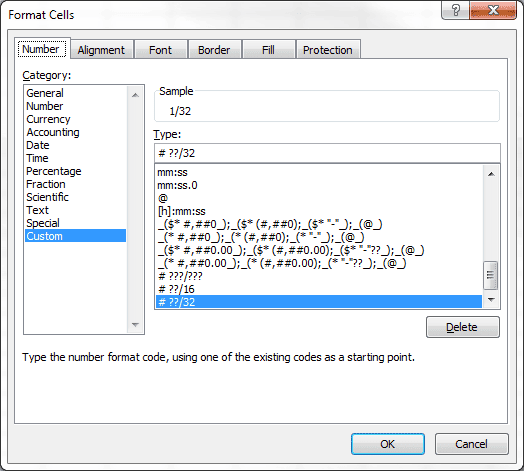# Figure Those Pesky Fractions in Excel

You’ve probably seen a conversion chart that takes US fractions and converts them to a decimal equivalent.

However, when you need to know the decimal equivalent of 7/16 or 5/32, there’s no chart to be found. And if you’re working on a project that requires more than a few conversions, using a calculator or conversion app is time consuming and frustrating.

Excel does fractions easily. In fact, creating your own custom chart is a simple matter.

## Enter a Fraction in Excel

To enter a fraction in Excel, enter the integer portion followed by a space, then the fraction. If the fraction is less than one (1) you have to enter a zero for the integer.

For example, if you enter 0 7/16 into a cell, it will show 7/16 and the formula bar will show the decimal fraction.

## Create a Fraction List

To create the fraction list shown above, I used the following steps.

1. Enter and format the headings (Fraction and Decimal)
2. Enter 0 1/16 in cell A2 (remember the space between zero and the fraction)
3. Enter 0 2/16 in cell A3 (fractions are reduced to their lowest common denominator)
4. Select A2:A3 then grab the fill handle in the lower right corner of A3 and pull down to fill in the series.
5. Select B2:B16. Enter an equals sign and select A2, then Ctrl + Enter to fill in the range.
6. Use keyboard shortcut Ctrl+Shift+tilde (~) to get the General format.

Three digit fractions didn’t work for me until I changed the Fraction on the Number tab of the Format Cells dialog box to Up to three digits.I was also able to re-format my list using the As sixteenths Fraction format, which changed all the fractions to sixteenths.

However, when I created a list of thirty seconds (32’s) there was no standard format to choose from, so I looked at the format for sixteenths, by clicking on the  Custom category, then changed it to # ??/32, which changed all fractions to thirty-seconds.## 2 thoughts on “Figure Those Pesky Fractions in Excel”

1.siboli

Hi
Can you make Excel work with fractions with more than 3 digits in the denominator?
Thanks

1.Gregory

@siboli, yes Excel does work with more than 3 digits, but you need to use a custom format. Go to the Format Cells dialog box (Ctrl + 1) and select the Number tab, then select Custom, and in the Type box enter # ????/???? which will give you thousands in the denominator.